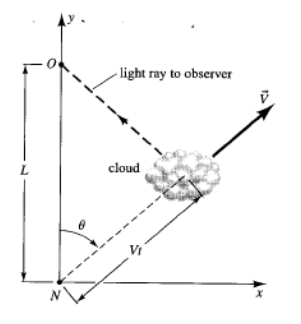## Wednesday 11 April 2018

### Exercise 1.04 Super luminal effects

#### The question was

Projection effects can trick you into thinking that an astrophysical object is moving “superluminally”. Consider a quasar that ejects gas with speed v at an angle θ with respect to the line-of-sight of the observer. Projected onto the sky, the gas appears to travel perpendicular to the line of sight with angular speed vapp/D, where D is the distance to the quasar and vapp is the apparent speed. Derive an expression for vapp in terms of v and θ. Show that, for appropriate values of v and θ, vapp can be greater than 1.

PSR J0108-1431, the closest known pulsar to the Earth, is 280 light years distant . If the ejected gas position was measured at times a year apart, the angular difference in position α would be 1/128 = 0.0078125. We remember that sin's and tan's of very small angles are very close to the angle.
I don't know why we have to bring relativity into this, so I haven't. Consider the diagram below, α is unrealistically big for visibility.

Our famous observer is at O and the pulsar is at P. The ejected gas arrives at G after, say a year, and it's velocity v is represented by PG. The apparent velocity vapp isis represented by the line PGapp. We added the line PQ at right angles to OGapp and passing thru P. By simple geometry, we get angles in this order:
Starting at O: PGO = π - α - θ then QGP = α + θ
Starting at Gapp: PGappO = π/2 - α then QPGapp = α
calculating  PQ in two different ways we get
PQ = vapp cos α   = v sin (α + θ)
Therefore
vapp   = v sin (α + θ) / cos α
This is not quite what was wanted, but for maximum leverage we obviously want v = π/2 - α so v lies along PQ. We then get
vapp   = v / cos α

Let's assume the gas comes from PSR J0108-1431 and that it's velocity is 0.99997, vapp is 1.000000517. If v is 0.99996, vapp is 0.999990517. It's a close run thing.

#### Embarassing

Sadly my answer was completely wrong as I discovered when I looked at the UCSB answer, which was very clear. They have a slightly different illustration (from Hartle). O is the Observer again and N is the pulsar. They also do not use relativity but they do take account of the fact that if the cloud is moving rapidly towards the observer, the time taken for the light to arrive will be shorter, making the apparent velocity larger. That was at least one of my mistakes.From Hartle via UCSB
The formula they arrive at is
vapp   = v sin θ / (1 - v cos θ)
I have omitted a c because, for us, it is always 1.

This gives much more exciting results. For example at v=0.99, the apparent velocity can reach nearly 7 times the speed of light! Here is a graph of vapp vs θ for a more modest v = 0.95.vapp vs θ for v = 0.95
1.2.